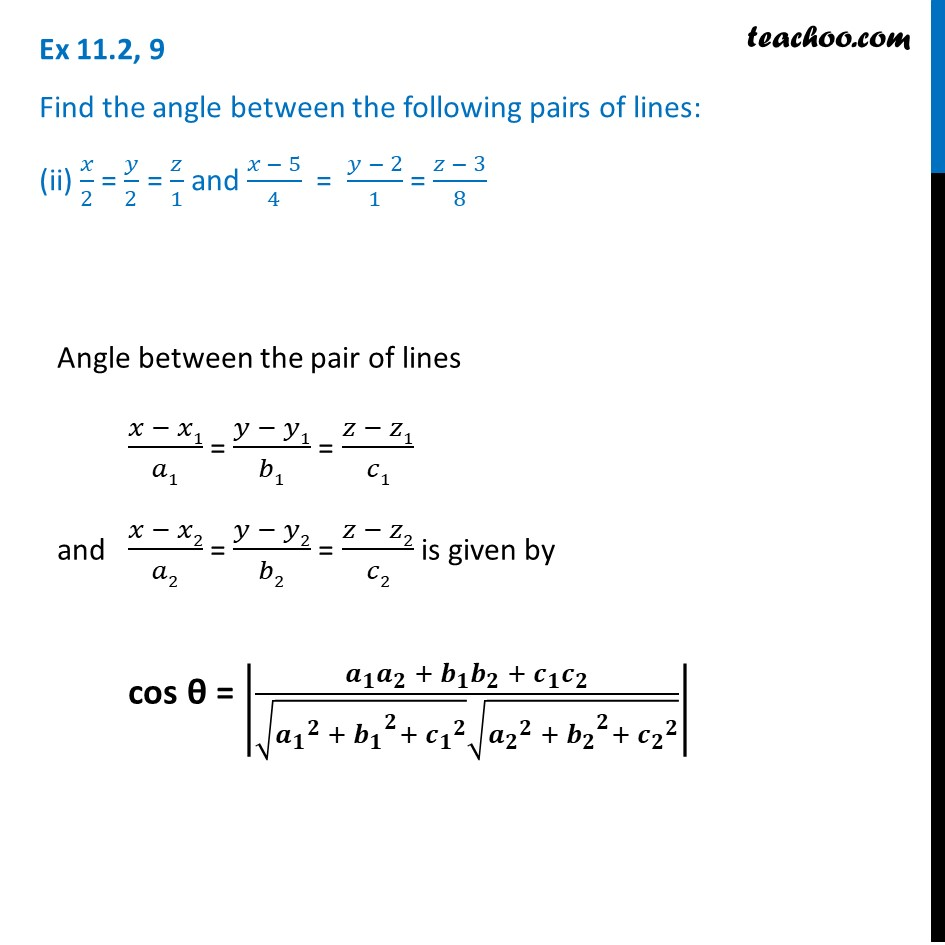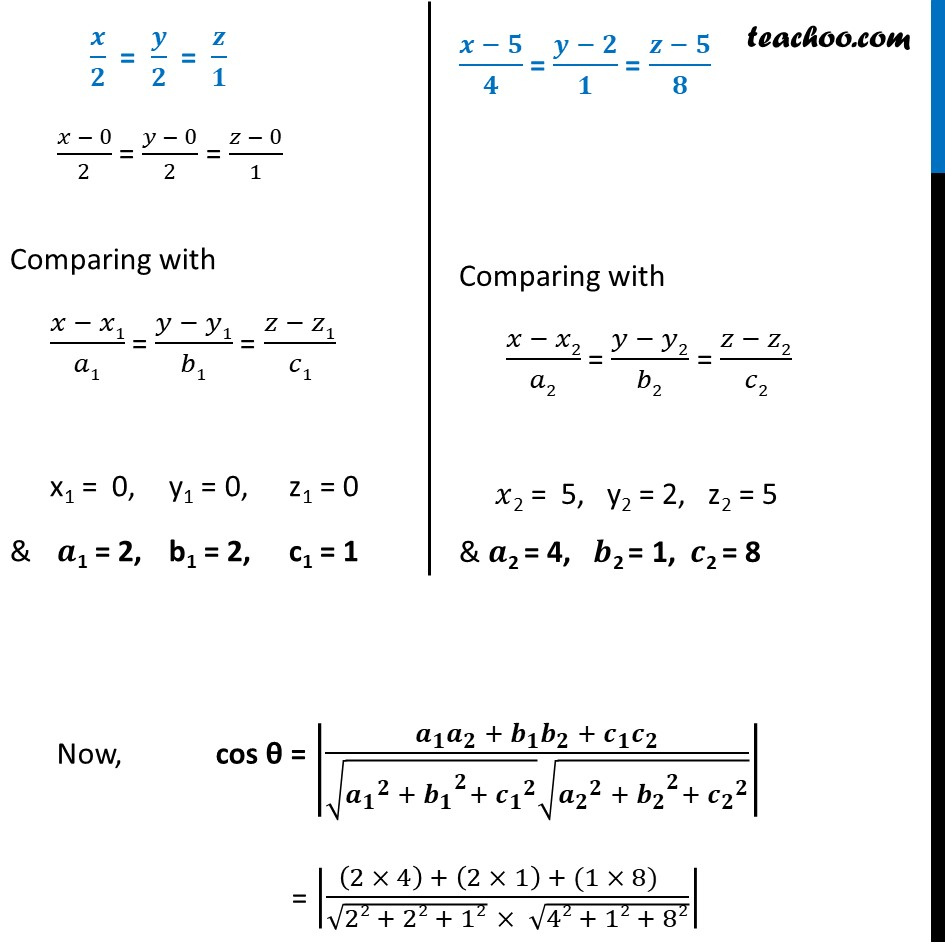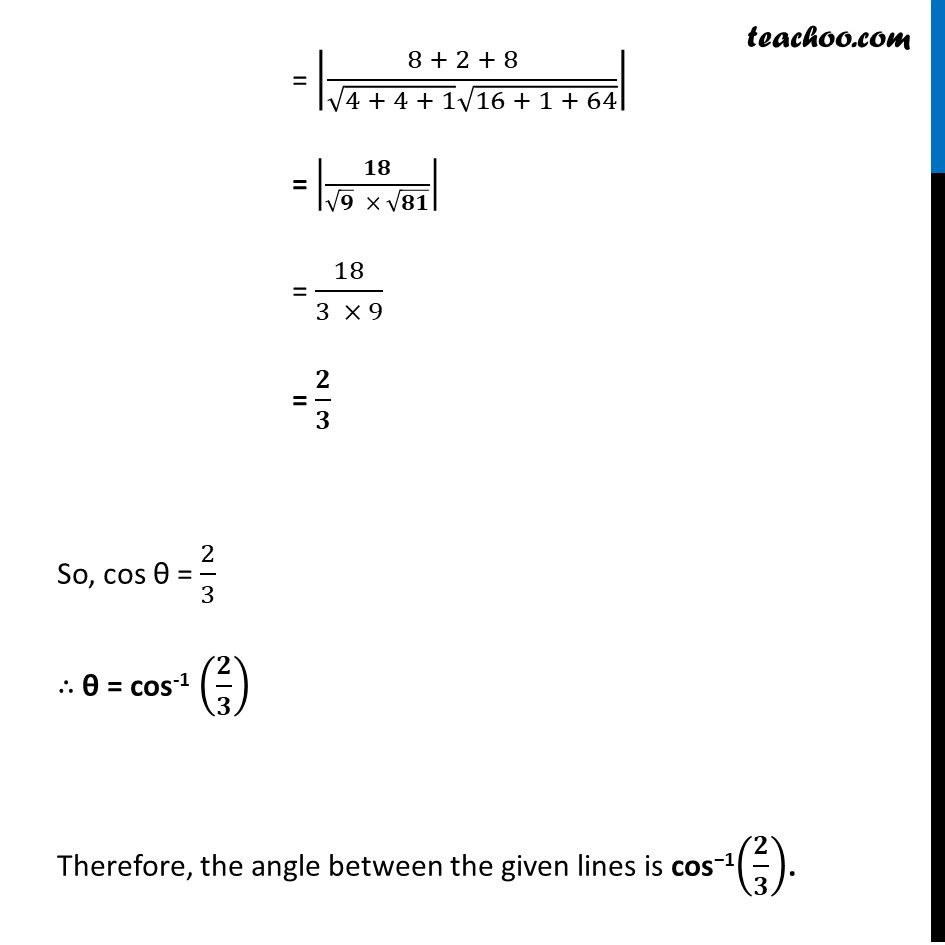Ex 11.2

Chapter 11 Class 12 Three Dimensional Geometry
Serial order wiseLearn in your speed, with individual attention - Teachoo Maths 1-on-1 Class

### Transcript

Ex 11.2, 9 Find the angle between the following pairs of lines: (ii) 𝑥/2 = 𝑦/2 = 𝑧/1 and (𝑥 − 5)/4 = (𝑦 − 2)/1 = (𝑧 − 3)/8Angle between the pair of lines (𝑥 − 𝑥1)/𝑎1 = (𝑦 − 𝑦1)/𝑏1 = (𝑧 − 𝑧1)/𝑐1 and (𝑥 − 𝑥2)/𝑎2 = (𝑦 − 𝑦2)/𝑏2 = (𝑧 − 𝑧2)/𝑐2 is given by cos θ = |(𝒂_𝟏 𝒂_𝟐 + 𝒃_𝟏 𝒃_𝟐 +〖 𝒄〗_𝟏 𝒄_𝟐)/(√(〖𝒂_𝟏〗^𝟐 + 〖𝒃_𝟏〗^𝟐+ 〖𝒄_𝟏〗^𝟐 ) √(〖𝒂_𝟐〗^𝟐 +〖〖 𝒃〗_𝟐〗^𝟐+ 〖𝒄_𝟐〗^𝟐 ))| 𝒙/𝟐 = 𝒚/𝟐 = 𝒛/𝟏 (𝑥 − 0)/2 = (𝑦 − 0)/2 = (𝑧 − 0)/1 Comparing with (𝑥 − 𝑥1)/𝑎1 = (𝑦 − 𝑦1)/𝑏1 = (𝑧 − 𝑧1)/𝑐1 x1 = 0, y1 = 0, z1 = 0 & 𝒂1 = 2, b1 = 2, c1 = 1 (𝒙 − 𝟓)/𝟒 = (𝒚 − 𝟐)/𝟏 = (𝒛 − 𝟓)/𝟖 Comparing with (𝑥 − 𝑥2)/𝑎2 = (𝑦 − 𝑦2)/𝑏2 = (𝑧 − 𝑧2)/𝑐2 𝑥2 = 5, y2 = 2, z2 = 5 & 𝒂2 = 4, 𝒃2 = 1, 𝒄2 = 8 Now, cos θ = |(𝒂_𝟏 𝒂_𝟐 + 𝒃_𝟏 𝒃_𝟐 +〖 𝒄〗_𝟏 𝒄_𝟐)/(√(〖𝒂_𝟏〗^𝟐 + 〖𝒃_𝟏〗^𝟐+ 〖𝒄_𝟏〗^𝟐 ) √(〖𝒂_𝟐〗^𝟐 +〖〖 𝒃〗_𝟐〗^𝟐+ 〖𝒄_𝟐〗^𝟐 ))| = |((2 × 4) + (2 × 1) + (1 × 8) )/(√(22 + 22 + 12) × √(42 + 12 + 82))| = |(8 + 2 + 8 )/(√(4 + 4 + 1) √(16 + 1 + 64))| = |𝟏𝟖/(√𝟗 × √𝟖𝟏)| = 18/(3 × 9) = 𝟐/𝟑 So, cos θ = 2/3 ∴ θ = cos-1 (𝟐/𝟑) Therefore, the angle between the given lines is cos−1(𝟐/𝟑).